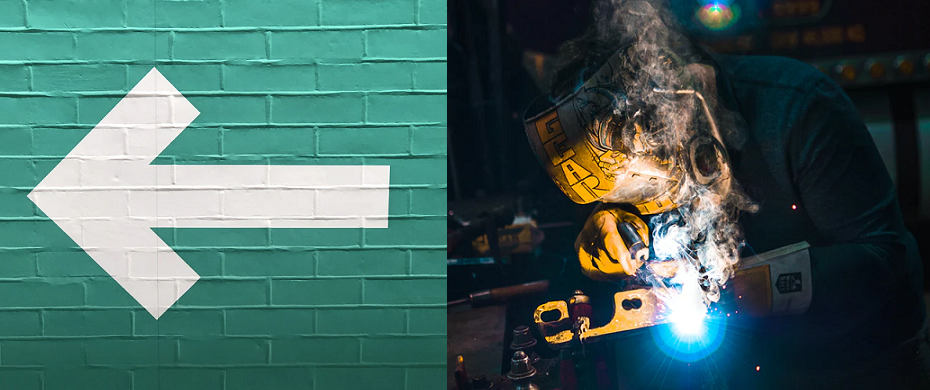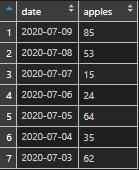# Left join only selected columns in RIf you want to use dplyr left join or any other type of join in R to combine information from two or multiple data frames, this post might be very helpful. Here is how to left join only selected columns in R.

The first data frame.

```first_df <- data.frame("date" = Sys.Date() - 1:7,
"apples" = floor(runif(7, min = 0, max = 101)))```The second data frame.

```second_df <- data.frame("date" = Sys.Date() - 1:7,
"elephants" = floor(runif(7, min = 0, max = 101)),
"bananas" = floor(runif(7, min = 0, max = 101)),
"cats" = floor(runif(7, min = 0, max = 101)))```How to perform dplyr left join and keep only necessary columns from the second data frame? In this case, let’s keep only elephants and cats.

To do that, use the select function that defines what comes from the second data frame.

Here are two different ways of how to do that.

```# first example
require(dplyr)

new_df <-
left_join(first_df,
second_df %>% dplyr::select(date, elephants, cats),
by = "date")

# second example
require(dplyr)

new_df <-
left_join(first_df,
second_df %>% dplyr::select(-bananas),
by = "date")```

Here is another post that might be useful in your toolbox – multiple left joins in R.